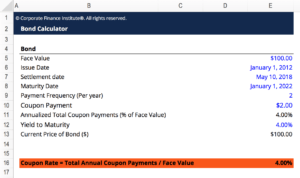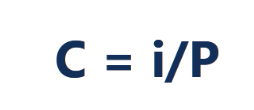# Coupon Rate Template

## Coupon Rate Template

This coupon rate template will calculate a bonds coupon rate based on the total annual coupon payments and the face value of the bond. As is customary with CFI templates the blue values are hardcoded numbers and black numbers are calculations dependent on other cells.

Here is a snippet of the template:### Coupon Rate Formula

The formula for calculating the Coupon Rate is as follows:Where:

C = Coupon rate

I = Annualized interest

P = Par value, or principal amount, of the bond

### More Free Templates

For more resources, check out our business templates library to download numerous free Excel modeling, PowerPoint presentation and Word document templates.

• Excel Modeling Templates
• PowerPoint Presentation Templates
• Transaction Document Templates